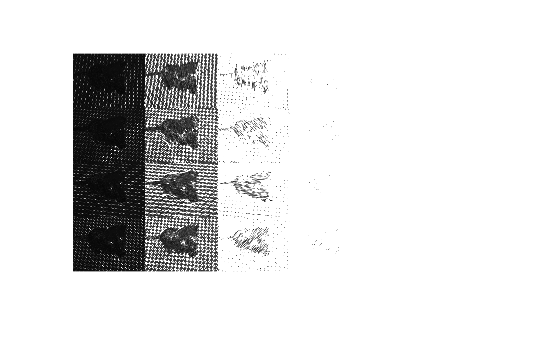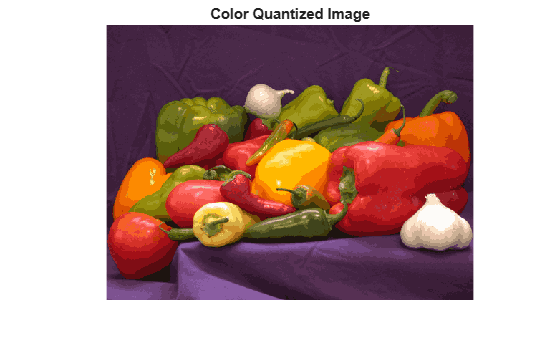# imsegkmeans

K-means clustering based image segmentation

## Syntax

``L = imsegkmeans(I,k)``
``[L,centers] = imsegkmeans(I,k)``
``L = imsegkmeans(I,k,Name,Value)``

## Description

example

````L = imsegkmeans(I,k)` segments image `I` into `k` clusters by performing k-means clustering and returns the segmented labeled output in `L`.```

example

````[L,centers] = imsegkmeans(I,k)` also returns the cluster centroid locations, `centers`.```
````L = imsegkmeans(I,k,Name,Value)` uses name-value arguments to control aspects of the k-means clustering algorithm.```

## Examples

collapse all

Read an image into the workspace.

```I = imread('cameraman.tif'); imshow(I) title('Original Image')```Segment the image into three regions using k-means clustering.

```[L,Centers] = imsegkmeans(I,3); B = labeloverlay(I,L); imshow(B) title('Labeled Image')```Read an image into the workspace. Reduce the image size to make the example run more quickly.

```RGB = imread('kobi.png'); RGB = imresize(RGB,0.5); imshow(RGB)```Segment the image into two regions using k-means clustering.

```L = imsegkmeans(RGB,2); B = labeloverlay(RGB,L); imshow(B) title('Labeled Image')```Several pixels are mislabeled. The rest of the example shows how to improve the k-means segmentation by supplementing the information about each pixel.

Supplement the image with information about the texture in the neighborhood of each pixel. To obtain the texture information, filter a grayscale version of the image with a set of Gabor filters.

Create a set of 24 Gabor filters, covering 6 wavelengths and 4 orientations.

```wavelength = 2.^(0:5) * 3; orientation = 0:45:135; g = gabor(wavelength,orientation);```

Convert the image to grayscale.

`I = rgb2gray(im2single(RGB));`

Filter the grayscale image using the Gabor filters. Display the 24 filtered images in a montage.

```gabormag = imgaborfilt(I,g); montage(gabormag,'Size',[4 6])```Smooth each filtered image to remove local variations. Display the smoothed images in a montage.

```for i = 1:length(g) sigma = 0.5*g(i).Wavelength; gabormag(:,:,i) = imgaussfilt(gabormag(:,:,i),3*sigma); end montage(gabormag,'Size',[4 6])```Supplement the information about each pixel with spatial location information. This additional information allows the k-means clustering algorithm to prefer groupings that are close together spatially.

Get the x and y coordinates of all pixels in the input image.

```nrows = size(RGB,1); ncols = size(RGB,2); [X,Y] = meshgrid(1:ncols,1:nrows);```

Concatenate the intensity information, neighborhood texture information, and spatial information about each pixel.

For this example, the feature set includes intensity image `I` instead of the original color image, `RGB`. The color information is omitted from the feature set because the yellow color of the dog's fur is similar to the yellow hue of the tiles. The color channels do not provide enough distinct information about the dog and the background to make a clean segmentation.

`featureSet = cat(3,I,gabormag,X,Y);`

Segment the image into two regions using k-means clustering with the supplemented feature set.

```L2 = imsegkmeans(featureSet,2,'NormalizeInput',true); C = labeloverlay(RGB,L2); imshow(C) title('Labeled Image with Additional Pixel Information')```Read an image into the workspace.

```I = imread('peppers.png'); imshow(I) title('Original Image')```Segment the image into 50 regions by using k-means clustering. Return the label matrix `L` and the cluster centroid locations `C`. The cluster centroid locations are the RGB values of each of the 50 colors.

`[L,C] = imsegkmeans(I,50);`

Convert the label matrix into an RGB image. Specify the cluster centroid locations, `C`, as the colormap for the new image.

`J = label2rgb(L,im2double(C));`

Display the quantized image.

```imshow(J) title('Color Quantized Image')```Write the original and compressed images to file. The quantized image file is approximate one quarter the size of the original image file.

```imwrite(I,'peppersOriginal.png'); imwrite(J,'peppersQuantized.png');```

## Input Arguments

collapse all

Image to segment, specified as a 2-D grayscale image, 2-D color image, or 2-D multispectral image.

Data Types: `single` | `int8` | `int16` | `uint8` | `uint16`

Number of clusters to create, specified as a positive integer.

### Name-Value Pair Arguments

Specify optional comma-separated pairs of `Name,Value` arguments. `Name` is the argument name and `Value` is the corresponding value. `Name` must appear inside quotes. You can specify several name and value pair arguments in any order as `Name1,Value1,...,NameN,ValueN`.

Example: `L = imsegkmeans(I,5,'NumAttempts',5);`

Normalize input data to zero mean and unit variance, specified as the comma-separated pair consisting of `'NormalizeInput'` and `true` or `false`. If you specify `true`, then `imsegkmeans` normalizes each channel of the input individually.

Number of times to repeat the clustering process using new initial cluster centroid positions, specified as the comma-separated pair consisting of `'NumAttempts'` and a positive integer.

Maximum number of iterations, specified as the comma-separated pair consisting of `'MaxIterations'` and a positive integer.

Accuracy threshold, specified as the comma-separated pair consisting of `'Threshold'` and a positive number. The algorithm stops when each of the cluster centers move less than the threshold value in consecutive iterations.

## Output Arguments

collapse all

Label matrix, specified as a matrix of positive integers. Pixels with label 1 belong to the first cluster, label 2 belong to the second cluster, and so on for each of the `k` clusters. `L` has the same first two dimensions as image `I`. The class of `L` depends on number of clusters.

Class of `L`Number of Clusters
`'uint8'``k <= 255`
`'uint16'``256 <= k <= 65535`
`'uint32'``65536 <= k <= 2^32-1`
`'double'``2^32 <= k`

Cluster centroid locations, returned as a numeric matrix of size k-by-c, where k is the number of clusters and c is the number of channels. `centers` is the same class as the image `I`.

## Tips

• The function yields reproducible results. The output will not vary in multiple runs given the same input arguments.

 Arthur, D. and S. Vassilvitskii. "k-means++: The Advantages of Careful Seeding." SODA '07: Proceedings of the Eighteenth Annual ACM-SIAM Symposium on Discrete Algorithms. New Orleans, LA, January 2007, pp. 1027–1035.